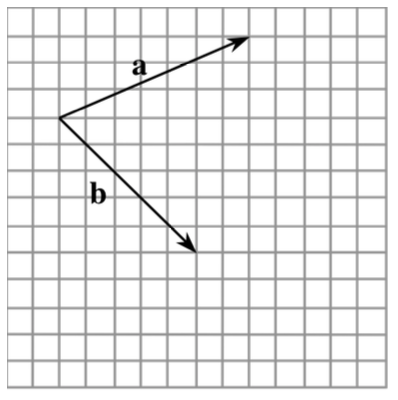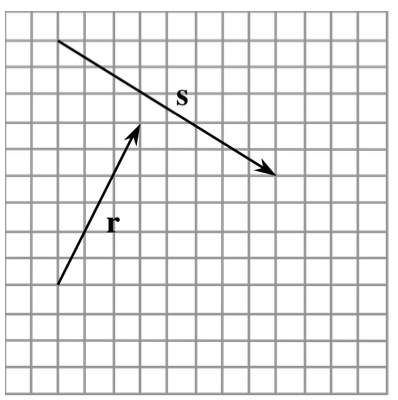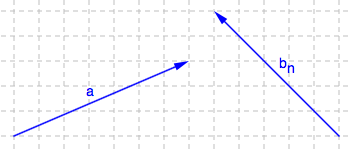### Home > PC > Chapter 10 > Lesson 10.1.2 > Problem10-21

10-21.

You know how to add vectors and you know how to draw the opposite of a given vector. You can use this knowledge to subtract vectors.1. Copy $\textbf{a}$ and $\textbf{b}$ onto graph paper. Draw $−\textbf{b}$. Then add $\textbf{a}$ and $−\textbf{b}$. Explain why you can label your answer $\textbf{a} − \textbf{b}$.

Vectors $\textbf{a}$ and $−\textbf{b}$ are sketched for you.
Reposition them so that you can draw the resultant vector.2. Copy $\mathbf{r}$ and $\mathbf{s}$ onto graph paper. Then draw $\mathbf{r} − \mathbf{s}$.